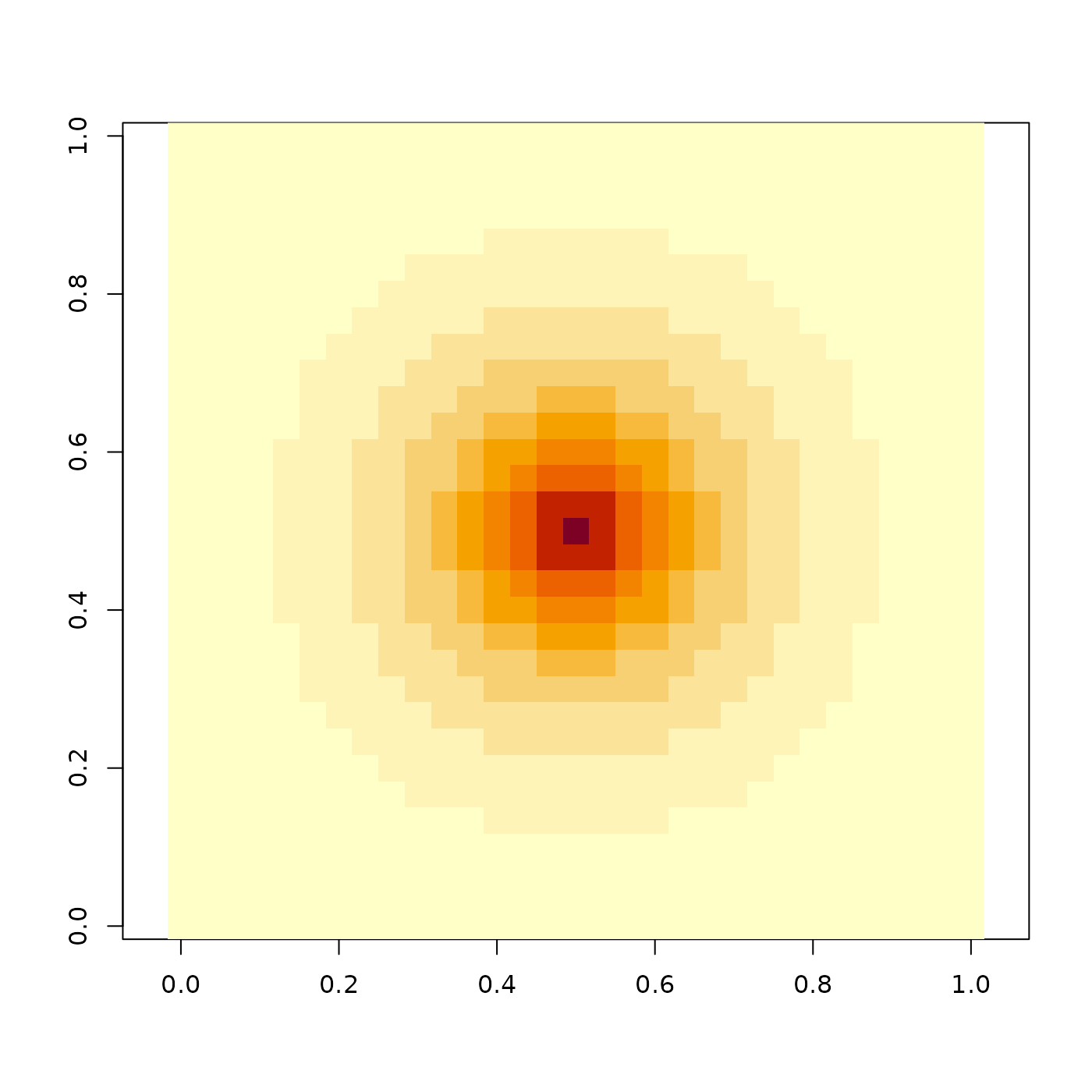Generates a 2D exponential distribution, with an optional argument to take the exponential to a user-defined power.

generate_2d_exponential(
falloff = 1,
dim = c(11, 11),
width = 3,
rescale_unity = FALSE
)

## Arguments

falloff

Default 1. Falloff of the exponential.

dim

Default c(11, 11). The dimensions of the matrix.

width

Default 3 (-10 to 10). The range in which to compute the distribution.

rescale_unity

Default FALSE. If TRUE, this will rescale the max value to one. Useful if wanting to plot the distribution with plot_image().

## Examples

if(rayimage:::run_documentation()){
image(generate_2d_exponential(1,31,3), asp=1)
}